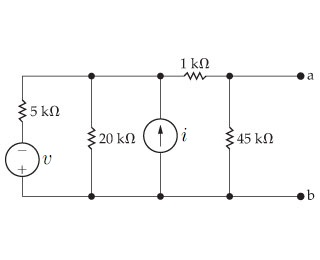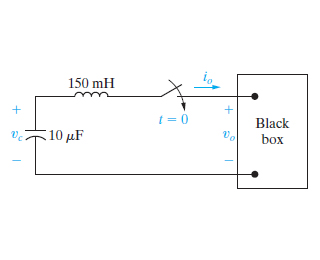Create an Account

Home / Questions / A voltmeter with a resistance of 92k is used to measure the voltage vab in the circuit Fig...

A voltmeter with a resistance of 92k is used to measure the voltage vab in the circuit Figure 1 1 What is the voltmeter reading if

A voltmeter with a resistance of 92k? is used to measure the voltage vab in the circuit.(Figure 1)1) What is the voltmeter reading if v = 48V and i = 17.6mA ?

2)What is the deviation percentage in the voltmeter reading if the percentage of deviation is defined as [(measured?actual)/actual]A????1100?

-----------------------------------------------------------------------------------

At t=0, a series-connected capacitor and inductor are placed across the terminals of a black box, as shown in the (Figure 1) . For t>0, it is known that io=200e?800t?40e?200tmA, where t is in seconds. vc(0) = 5 V.1Find vo for t >= 0. answer in terms of t, where t is in milimeters

Show transcribed image text A voltmeter with a resistance of 92k? is used to measure the voltage vab in the circuit.(Figure 1) 1Find vo for t >= 0. answer in terms of t, where t is in milimeters 1) What is the voltmeter reading if v = 48V and i = 17.6mA ? 1) What is the voltmeter reading if v = 48V and i = 17.6mA ? 2)What is the deviation percentage in the voltmeter reading if the percentage of deviation is defined as [(measured?actual)/actual]A???A????100? ----------------------------------------------------------------------------------- At t=0, a series-connected capacitor and inductor are placed across the terminals of a black box, as shown in the (Figure 1) . For t>0, it is known that io=200e?800t?40e?200tmA, where t is in seconds. vc(0) = 5 V.

Jul 27 2020 View more View LessSubscribe To Get Solution